Next: Constraints for the vector-isovector Up: Continuity equations in the Previous: Constraints for the scalar-isovector

## Constraints for the vector-isoscalar channel

Validity of the CE for the vector-isoscalar density, Eq. (30) for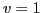and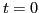, imposes through Eq. (48) at second order the following constraints on the coupling constants of the functional,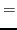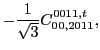(59)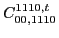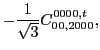(60)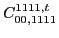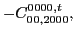(61)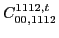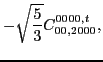(62)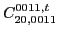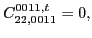(63)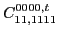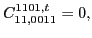(64)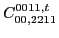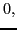(65)

whereas the two coupling constants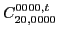are left unrestricted. We note here that the constraints now connect scalar and vector coupling constants. Altogether, at second order, for the vector-isoscalar channel of the CE we have 6 free and 8 dependent coupling constants. Apart from that, 10 second-order coupling constants must vanish, which includes the surface ones in Eq. (63), spin-orbit ones of the Eq. (64), and tensor ones in Eq. (65).

For the fourth and sixth orders, analogous constraints are presented in Appendix B.Next: Constraints for the vector-isovector Up: Continuity equations in the Previous: Constraints for the scalar-isovector
Jacek Dobaczewski 2011-11-11